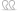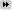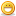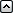# Thema: "Meinten Sie [...]" in Python?

1. ##AW: "Meinten Sie [...]" in Python?Zitat von Jut4h.tmwenn du möchtest kann ich den code mal posten (wenn du noch Hilfe brauchst)
Ich würde den Code gerne einmal sehen. Hab in diese Richtung bisher nämlich garnix gemacht.Habe mich jetzt auch einmal dran gesetzt und nachfolgender Code ist dabei rausgekommen. Bitte seid gnädig mit mir, ich entwickle eigentlich nicht in Python und hab das nur in Python gemacht, weil das ja so gefordert war. Also ich habe bestimmt einige Stilfehler drin.Zum Code:
Der Code fragt direkt bei GooglePlay die Package-Namen der Apps. Das Ergebnis ist selbstverständlich abhängig von dem Suchparameter. Diesen könnt ihr anpassen, in dem ihr den Inhalt der Variable "QUERY" (ganz oben) einmal anpasst. Im Moment steht "the+room" drin (im übrigen ein top Spiel). Leerzeichen müssen durch ein "+" gekennzeichnet werden.

Ich habe mir Mühe gegeben den Code zu verständlich wie nur möglich zu kommentieren. Aber kurz zusammengefasst rufe ich GooglePlay mit genau den Suchparamtern auf und parse mir aus der Antwort alle Links heraus ("href="). Diese verweisen weiter auf die Detailseiten der Apps, und der Link enthält den Package-Namen. Schön ist hierbei auch, dass so durch kleine Anpassungen auch der Kiosk und die Filme druchsucht werden können. Derzeit wird aber nur nach Apps gesucht. Hier also der Code:

Viele Grüße,

BarnyZitieren

2. ### Folgende Benutzer haben sich für diesen Beitrag bedankt:

Dailox (03.12.2016), Starflow (18.11.2016)

3. ##AW: "Meinten Sie [...]" in Python?

Danke für alle eure Antworten! Barnys Lösung hat mir sehr weitergeholfen.

sry, dass ich nicht sofort geantwortet hatte. Rl und so kam dazwischen ^^Zitieren

4. ##AW: "Meinten Sie [...]" in Python?

Hey,

hab mich auch eben rangesetzt und den von Barny bereitgestellten Code (danke dafür) nochmals refactored.
Mit regulären Ausdrücken konnte man einiges an Zeilen sparen. 8)

Code:
```# -*- encoding: utf-8 -*-
import urllib.request
import re
from functools import reduce

# Must-Have Data
QUERY = "the+room"

# Hole den ganzen HTML-Geschiss von GooglePlay

# Hole alle URL´s aus dem Quelltext heraus
def parse_urls(content):
regex = re.compile(r"href=\"(.*?)\"")
return regex.findall(content)

# Holt aus den geparsten URL´s die Namen der Pakete heraus
def parse_app_names(app_list):
all_as_str = reduce(lambda x, y: x + " " + y, app_list)
regex = re.compile(r"/store/apps/details\?id=(.+?) ")
return list(set(regex.findall(all_as_str)))

# Hole URL´s

# Parse aus den URL´s die Paketnamen
app_names = parse_app_names(urls)

# Gib die ganze Liste aus
for name in app_names:
print(name)```
Gruss,
sp1nnyZitieren

5. ### Folgende Benutzer haben sich für diesen Beitrag bedankt:

Barny (06.12.2016), Dailox (07.12.2016)

6. ##AW: "Meinten Sie [...]" in Python?

Hier ist der SQL Code. Man nutzt die Funktion indem man heuristicScore([DIE SPALTE DIE ABGEFRAGT WERDEN SOLL],[DER STRING DEN MAN SUCHT]) mit in den query packt. Die Funktion haut eine zahl raus. Je höher die zahl ist desto wahrscheinlicher ist das Wort gemeint. man muss die liste dann nur noch nach der score sortieren.

Code:
```DELIMITER \$\$

DROP FUNCTION IF EXISTS `flb`.`dm` \$\$
CREATE FUNCTION `dm`(st VARCHAR(55)) RETURNS varchar(128) CHARSET utf8
NO SQL
BEGIN
DECLARE length, first, last, pos, prevpos, is_slavo_germanic SMALLINT;
DECLARE pri, sec VARCHAR(45) DEFAULT '';
DECLARE ch CHAR(1);
SET first = 3;
SET length = CHAR_LENGTH(st);
SET last = first + length -1;
SET st = CONCAT(REPEAT('-', first -1), UCASE(st), REPEAT(' ', 5));
SET is_slavo_germanic = (st LIKE '%W%' OR st LIKE '%K%' OR st LIKE '%CZ%');
SET pos = first;
IF SUBSTRING(st, first, 2) IN ('GN', 'KN', 'PN', 'WR', 'PS') THEN
SET pos = pos + 1;
END IF;
IF SUBSTRING(st, first, 1) = 'X' THEN
SET pri = 'S', sec = 'S', pos = pos  + 1;
END IF;
WHILE pos <= last DO
SET prevpos = pos;
SET ch = SUBSTRING(st, pos, 1);
CASE
WHEN ch IN ('A', 'E', 'I', 'O', 'U', 'Y') THEN
IF pos = first THEN
SET pri = CONCAT(pri, 'A'), sec = CONCAT(sec, 'A'), pos = pos  + 1;
ELSE
SET pos = pos + 1;
END IF;
WHEN ch = 'B' THEN

IF SUBSTRING(st, pos+1, 1) = 'B' THEN
SET pri = CONCAT(pri, 'P'), sec = CONCAT(sec, 'P'), pos = pos  + 2;
ELSE
SET pri = CONCAT(pri, 'P'), sec = CONCAT(sec, 'P'), pos = pos  + 1;
END IF;
WHEN ch = 'C' THEN

IF (pos > (first + 1) AND SUBSTRING(st, pos-2, 1) NOT IN ('A', 'E', 'I', 'O', 'U', 'Y') AND SUBSTRING(st, pos-1, 3) = 'ACH' AND
(SUBSTRING(st, pos+2, 1) NOT IN ('I', 'E') OR SUBSTRING(st, pos-2, 6) IN ('BACHER', 'MACHER'))) THEN
SET pri = CONCAT(pri, 'K'), sec = CONCAT(sec, 'K'), pos = pos  + 2;

ELSEIF pos = first AND SUBSTRING(st, first, 6) = 'CAESAR' THEN
SET pri = CONCAT(pri, 'S'), sec = CONCAT(sec, 'S'), pos = pos  + 2;
ELSEIF SUBSTRING(st, pos, 4) = 'CHIA' THEN
SET pri = CONCAT(pri, 'K'), sec = CONCAT(sec, 'K'), pos = pos  + 2;
ELSEIF SUBSTRING(st, pos, 2) = 'CH' THEN

IF pos > first AND SUBSTRING(st, pos, 4) = 'CHAE' THEN
SET pri = CONCAT(pri, 'K'), sec = CONCAT(sec, 'X'), pos = pos  + 2;
ELSEIF pos = first AND (SUBSTRING(st, pos+1, 5) IN ('HARAC', 'HARIS') OR
SUBSTRING(st, pos+1, 3) IN ('HOR', 'HYM', 'HIA', 'HEM')) AND SUBSTRING(st, first, 5) != 'CHORE' THEN
SET pri = CONCAT(pri, 'K'), sec = CONCAT(sec, 'K'), pos = pos  + 2;

ELSEIF SUBSTRING(st, first, 4) IN ('VAN ', 'VON ') OR SUBSTRING(st, first, 3) = 'SCH'
OR SUBSTRING(st, pos-2, 6) IN ('ORCHES', 'ARCHIT', 'ORCHID')
OR SUBSTRING(st, pos+2, 1) IN ('T', 'S')
OR ((SUBSTRING(st, pos-1, 1) IN ('A', 'O', 'U', 'E') OR pos = first)
AND SUBSTRING(st, pos+2, 1) IN ('L', 'R', 'N', 'M', 'B', 'H', 'F', 'V', 'W', ' ')) THEN
SET pri = CONCAT(pri, 'K'), sec = CONCAT(sec, 'K'), pos = pos  + 2;
ELSE
IF pos > first THEN
IF SUBSTRING(st, first, 2) = 'MC' THEN
SET pri = CONCAT(pri, 'K'), sec = CONCAT(sec, 'K'), pos = pos  + 2;
ELSE
SET pri = CONCAT(pri, 'X'), sec = CONCAT(sec, 'K'), pos = pos  + 2;
END IF;
ELSE
SET pri = CONCAT(pri, 'X'), sec = CONCAT(sec, 'X'), pos = pos  + 2;
END IF;
END IF;

ELSEIF SUBSTRING(st, pos, 2) = 'CZ' AND SUBSTRING(st, pos-2, 4) != 'WICZ' THEN
SET pri = CONCAT(pri, 'S'), sec = CONCAT(sec, 'X'), pos = pos  + 2;

ELSEIF SUBSTRING(st, pos+1, 3) = 'CIA' THEN
SET pri = CONCAT(pri, 'X'), sec = CONCAT(sec, 'X'), pos = pos  + 3;

ELSEIF SUBSTRING(st, pos, 2) = 'CC' AND NOT (pos = (first +1) AND SUBSTRING(st, first, 1) = 'M') THEN

IF SUBSTRING(st, pos+2, 1) IN ('I', 'E', 'H') AND SUBSTRING(st, pos+2, 2) != 'HU' THEN

IF (pos = first +1 AND SUBSTRING(st, first) = 'A') OR
SUBSTRING(st, pos-1, 5) IN ('UCCEE', 'UCCES') THEN
SET pri = CONCAT(pri, 'KS'), sec = CONCAT(sec, 'KS'), pos = pos  + 3;

ELSE
SET pri = CONCAT(pri, 'X'), sec = CONCAT(sec, 'X'), pos = pos  + 3;
END IF;
ELSE
SET pri = CONCAT(pri, 'K'), sec = CONCAT(sec, 'K'), pos = pos  + 2;
END IF;
ELSEIF SUBSTRING(st, pos, 2) IN ('CK', 'CG', 'CQ') THEN
SET pri = CONCAT(pri, 'K'), sec = CONCAT(sec, 'K'), pos = pos  + 2;
ELSEIF SUBSTRING(st, pos, 2) IN ('CI', 'CE', 'CY') THEN

IF SUBSTRING(st, pos, 3) IN ('CIO', 'CIE', 'CIA') THEN
SET pri = CONCAT(pri, 'S'), sec = CONCAT(sec, 'X'), pos = pos  + 2;
ELSE
SET pri = CONCAT(pri, 'S'), sec = CONCAT(sec, 'S'), pos = pos  + 2;
END IF;
ELSE

IF SUBSTRING(st, pos+1, 2) IN (' C', ' Q', ' G') THEN
SET pri = CONCAT(pri, 'K'), sec = CONCAT(sec, 'K'), pos = pos  + 3;
ELSE
IF SUBSTRING(st, pos+1, 1) IN ('C', 'K', 'Q') AND SUBSTRING(st, pos+1, 2) NOT IN ('CE', 'CI') THEN
SET pri = CONCAT(pri, 'K'), sec = CONCAT(sec, 'K'), pos = pos  + 2;
ELSE
SET pri = CONCAT(pri, 'K'), sec = CONCAT(sec, 'K'), pos = pos  + 1;
END IF;
END IF;
END IF;

WHEN ch = 'D' THEN
IF SUBSTRING(st, pos, 2) = 'DG' THEN
IF SUBSTRING(st, pos+2, 1) IN ('I', 'E', 'Y') THEN
SET pri = CONCAT(pri, 'J'), sec = CONCAT(sec, 'J'), pos = pos  + 3;
ELSE
SET pri = CONCAT(pri, 'TK'), sec = CONCAT(sec, 'TK'), pos = pos  + 2;
END IF;
ELSEIF SUBSTRING(st, pos, 2) IN ('DT', 'DD') THEN
SET pri = CONCAT(pri, 'T'), sec = CONCAT(sec, 'T'), pos = pos  + 2;
ELSE
SET pri = CONCAT(pri, 'T'), sec = CONCAT(sec, 'T'), pos = pos  + 1;
END IF;
WHEN ch = 'F' THEN
IF SUBSTRING(st, pos+1, 1) = 'F' THEN
SET pri = CONCAT(pri, 'F'), sec = CONCAT(sec, 'F'), pos = pos  + 2;
ELSE
SET pri = CONCAT(pri, 'F'), sec = CONCAT(sec, 'F'), pos = pos  + 1;
END IF;
WHEN ch = 'G' THEN
IF SUBSTRING(st, pos+1, 1) = 'H' THEN
IF (pos > first AND SUBSTRING(st, pos-1, 1) NOT IN ('A', 'E', 'I', 'O', 'U', 'Y'))
OR ( pos = first AND SUBSTRING(st, pos+2, 1) != 'I') THEN
SET pri = CONCAT(pri, 'K'), sec = CONCAT(sec, 'K'), pos = pos  + 2;
ELSEIF pos = first AND SUBSTRING(st, pos+2, 1) = 'I' THEN
SET pri = CONCAT(pri, 'J'), sec = CONCAT(sec, 'J'), pos = pos  + 2;

ELSEIF (pos > (first + 1) AND SUBSTRING(st, pos-2, 1) IN ('B', 'H', 'D') )
OR (pos > (first + 2) AND SUBSTRING(st, pos-3, 1) IN ('B', 'H', 'D') )
OR (pos > (first + 3) AND SUBSTRING(st, pos-4, 1) IN ('B', 'H') ) THEN
SET pos = pos + 2;
ELSE

IF pos > (first + 2) AND SUBSTRING(st, pos-1, 1) = 'U'
AND SUBSTRING(st, pos-3, 1) IN ('C', 'G', 'L', 'R', 'T') THEN
SET pri = CONCAT(pri, 'F'), sec = CONCAT(sec, 'F'), pos = pos  + 2;
ELSEIF pos > first AND SUBSTRING(st, pos-1, 1) != 'I' THEN
SET pri = CONCAT(pri, 'K'), sec = CONCAT(sec, 'K'), pos = pos  + 2;
ELSE
SET pos = pos + 1;
END IF;
END IF;
ELSEIF SUBSTRING(st, pos+1, 1) = 'N' THEN
IF pos = (first +1) AND SUBSTRING(st, first, 1) IN ('A', 'E', 'I', 'O', 'U', 'Y') AND NOT is_slavo_germanic THEN
SET pri = CONCAT(pri, 'KN'), sec = CONCAT(sec, 'N'), pos = pos  + 2;
ELSE

IF SUBSTRING(st, pos+2, 2) != 'EY' AND SUBSTRING(st, pos+1, 1) != 'Y'
AND NOT is_slavo_germanic THEN
SET pri = CONCAT(pri, 'N'), sec = CONCAT(sec, 'KN'), pos = pos  + 2;
ELSE
SET pri = CONCAT(pri, 'KN'), sec = CONCAT(sec, 'KN'), pos = pos  + 2;
END IF;
END IF;

ELSEIF SUBSTRING(st, pos+1, 2) = 'LI' AND NOT is_slavo_germanic THEN
SET pri = CONCAT(pri, 'KL'), sec = CONCAT(sec, 'L'), pos = pos  + 2;

ELSEIF pos = first AND (SUBSTRING(st, pos+1, 1) = 'Y'
OR SUBSTRING(st, pos+1, 2) IN ('ES', 'EP', 'EB', 'EL', 'EY', 'IB', 'IL', 'IN', 'IE', 'EI', 'ER')) THEN
SET pri = CONCAT(pri, 'K'), sec = CONCAT(sec, 'J'), pos = pos  + 2;

ELSEIF (SUBSTRING(st, pos+1, 2) = 'ER' OR SUBSTRING(st, pos+1, 1) = 'Y')
AND SUBSTRING(st, first, 6) NOT IN ('DANGER', 'RANGER', 'MANGER')
AND SUBSTRING(st, pos-1, 1) not IN ('E', 'I') AND SUBSTRING(st, pos-1, 3) NOT IN ('RGY', 'OGY') THEN
SET pri = CONCAT(pri, 'K'), sec = CONCAT(sec, 'J'), pos = pos  + 2;

ELSEIF SUBSTRING(st, pos+1, 1) IN ('E', 'I', 'Y') OR SUBSTRING(st, pos-1, 4) IN ('AGGI', 'OGGI') THEN

IF SUBSTRING(st, first, 4) IN ('VON ', 'VAN ') OR SUBSTRING(st, first, 3) = 'SCH'
OR SUBSTRING(st, pos+1, 2) = 'ET' THEN
SET pri = CONCAT(pri, 'K'), sec = CONCAT(sec, 'K'), pos = pos  + 2;
ELSE

IF SUBSTRING(st, pos+1, 4) = 'IER ' THEN
SET pri = CONCAT(pri, 'J'), sec = CONCAT(sec, 'J'), pos = pos  + 2;
ELSE
SET pri = CONCAT(pri, 'J'), sec = CONCAT(sec, 'K'), pos = pos  + 2;
END IF;
END IF;
ELSEIF SUBSTRING(st, pos+1, 1) = 'G' THEN
SET pri = CONCAT(pri, 'K'), sec = CONCAT(sec, 'K'), pos = pos  + 2;
ELSE
SET pri = CONCAT(pri, 'K'), sec = CONCAT(sec, 'K'), pos = pos  + 1;
END IF;
WHEN ch = 'H' THEN

IF (pos = first OR SUBSTRING(st, pos-1, 1) IN ('A', 'E', 'I', 'O', 'U', 'Y'))
AND SUBSTRING(st, pos+1, 1) IN ('A', 'E', 'I', 'O', 'U', 'Y') THEN
SET pri = CONCAT(pri, 'H'), sec = CONCAT(sec, 'H'), pos = pos  + 2;
ELSE
SET pos = pos + 1;
END IF;
WHEN ch = 'J' THEN

IF SUBSTRING(st, pos, 4) = 'JOSE' OR SUBSTRING(st, first, 4) = 'SAN ' THEN
IF (pos = first AND SUBSTRING(st, pos+4, 1) = ' ') OR SUBSTRING(st, first, 4) = 'SAN ' THEN
SET pri = CONCAT(pri, 'H'), sec = CONCAT(sec, 'H');
ELSE
SET pri = CONCAT(pri, 'J'), sec = CONCAT(sec, 'H');
END IF;
ELSEIF pos = first AND SUBSTRING(st, pos, 4) != 'JOSE' THEN
SET pri = CONCAT(pri, 'J'), sec = CONCAT(sec, 'A');
ELSE

IF SUBSTRING(st, pos-1, 1) IN ('A', 'E', 'I', 'O', 'U', 'Y') AND NOT is_slavo_germanic
AND SUBSTRING(st, pos+1, 1) IN ('A', 'O') THEN
SET pri = CONCAT(pri, 'J'), sec = CONCAT(sec, 'H');
ELSE
IF pos = last THEN
SET pri = CONCAT(pri, 'J');
ELSE
IF SUBSTRING(st, pos+1, 1) not IN ('L', 'T', 'K', 'S', 'N', 'M', 'B', 'Z')
AND SUBSTRING(st, pos-1, 1) not IN ('S', 'K', 'L') THEN
SET pri = CONCAT(pri, 'J'), sec = CONCAT(sec, 'J');
END IF;
END IF;
END IF;
END IF;
IF SUBSTRING(st, pos+1, 1) = 'J' THEN
SET pos = pos + 2;
ELSE
SET pos = pos + 1;
END IF;
WHEN ch = 'K' THEN
IF SUBSTRING(st, pos+1, 1) = 'K' THEN
SET pri = CONCAT(pri, 'K'), sec = CONCAT(sec, 'K'), pos = pos  + 2;
ELSE
SET pri = CONCAT(pri, 'K'), sec = CONCAT(sec, 'K'), pos = pos  + 1;
END IF;
WHEN ch = 'L' THEN
IF SUBSTRING(st, pos+1, 1) = 'L' THEN

IF (pos = (last - 2) AND SUBSTRING(st, pos-1, 4) IN ('ILLO', 'ILLA', 'ALLE'))
OR ((SUBSTRING(st, last-1, 2) IN ('AS', 'OS') OR SUBSTRING(st, last) IN ('A', 'O'))
AND SUBSTRING(st, pos-1, 4) = 'ALLE') THEN
SET pri = CONCAT(pri, 'L'), pos = pos  + 2;
ELSE
SET pri = CONCAT(pri, 'L'), sec = CONCAT(sec, 'L'), pos = pos  + 2;
END IF;
ELSE
SET pri = CONCAT(pri, 'L'), sec = CONCAT(sec, 'L'), pos = pos  + 1;
END IF;
WHEN ch = 'M' THEN
IF SUBSTRING(st, pos-1, 3) = 'UMB'
AND (pos + 1 = last OR SUBSTRING(st, pos+2, 2) = 'ER')
OR SUBSTRING(st, pos+1, 1) = 'M' THEN
SET pri = CONCAT(pri, 'M'), sec = CONCAT(sec, 'M'), pos = pos  + 2;
ELSE
SET pri = CONCAT(pri, 'M'), sec = CONCAT(sec, 'M'), pos = pos  + 1;
END IF;
WHEN ch = 'N' THEN
IF SUBSTRING(st, pos+1, 1) = 'N' THEN
SET pri = CONCAT(pri, 'N'), sec = CONCAT(sec, 'N'), pos = pos  + 2;
ELSE
SET pri = CONCAT(pri, 'N'), sec = CONCAT(sec, 'N'), pos = pos  + 1;
END IF;

WHEN ch = 'P' THEN
IF SUBSTRING(st, pos+1, 1) = 'H' THEN
SET pri = CONCAT(pri, 'F'), sec = CONCAT(sec, 'F'), pos = pos  + 2;
ELSEIF SUBSTRING(st, pos+1, 1) IN ('P', 'B') THEN
SET pri = CONCAT(pri, 'P'), sec = CONCAT(sec, 'P'), pos = pos  + 2;
ELSE
SET pri = CONCAT(pri, 'P'), sec = CONCAT(sec, 'P'), pos = pos  + 1;
END IF;
WHEN ch = 'Q' THEN
IF SUBSTRING(st, pos+1, 1) = 'Q' THEN
SET pri = CONCAT(pri, 'K'), sec = CONCAT(sec, 'K'), pos = pos  + 2;
ELSE
SET pri = CONCAT(pri, 'K'), sec = CONCAT(sec, 'K'), pos = pos  + 1;
END IF;
WHEN ch = 'R' THEN

IF pos = last AND not is_slavo_germanic
AND SUBSTRING(st, pos-2, 2) = 'IE' AND SUBSTRING(st, pos-4, 2) NOT IN ('ME', 'MA') THEN
SET sec = CONCAT(sec, 'R');
ELSE
SET pri = CONCAT(pri, 'R'), sec = CONCAT(sec, 'R');
END IF;
IF SUBSTRING(st, pos+1, 1) = 'R' THEN
SET pos = pos + 2;
ELSE
SET pos = pos + 1;
END IF;
WHEN ch = 'S' THEN

IF SUBSTRING(st, pos-1, 3) IN ('ISL', 'YSL') THEN
SET pos = pos + 1;

ELSEIF pos = first AND SUBSTRING(st, first, 5) = 'SUGAR' THEN
SET pri = CONCAT(pri, 'X'), sec = CONCAT(sec, 'S'), pos = pos  + 1;
ELSEIF SUBSTRING(st, pos, 2) = 'SH' THEN

IF SUBSTRING(st, pos+1, 4) IN ('HEIM', 'HOEK', 'HOLM', 'HOLZ') THEN
SET pri = CONCAT(pri, 'S'), sec = CONCAT(sec, 'S'), pos = pos  + 2;
ELSE
SET pri = CONCAT(pri, 'X'), sec = CONCAT(sec, 'X'), pos = pos  + 2;
END IF;

ELSEIF SUBSTRING(st, pos, 3) IN ('SIO', 'SIA') OR SUBSTRING(st, pos, 4) = 'SIAN' THEN
IF NOT is_slavo_germanic THEN
SET pri = CONCAT(pri, 'S'), sec = CONCAT(sec, 'X'), pos = pos  + 3;
ELSE
SET pri = CONCAT(pri, 'S'), sec = CONCAT(sec, 'S'), pos = pos  + 3;
END IF;

ELSEIF (pos = first AND SUBSTRING(st, pos+1, 1) IN ('M', 'N', 'L', 'W')) OR SUBSTRING(st, pos+1, 1) = 'Z' THEN
SET pri = CONCAT(pri, 'S'), sec = CONCAT(sec, 'X');
IF SUBSTRING(st, pos+1, 1) = 'Z' THEN
SET pos = pos + 2;
ELSE
SET pos = pos + 1;
END IF;
ELSEIF SUBSTRING(st, pos, 2) = 'SC' THEN

IF SUBSTRING(st, pos+2, 1) = 'H' THEN

IF SUBSTRING(st, pos+3, 2) IN ('OO', 'ER', 'EN', 'UY', 'ED', 'EM') THEN

IF SUBSTRING(st, pos+3, 2) IN ('ER', 'EN') THEN
SET pri = CONCAT(pri, 'X'), sec = CONCAT(sec, 'SK'), pos = pos  + 3;
ELSE
SET pri = CONCAT(pri, 'SK'), sec = CONCAT(sec, 'SK'), pos = pos  + 3;
END IF;
ELSE
IF pos = first AND SUBSTRING(st, first+3, 1) not IN ('A', 'E', 'I', 'O', 'U', 'Y') AND SUBSTRING(st, first+3, 1) != 'W' THEN
SET pri = CONCAT(pri, 'X'), sec = CONCAT(sec, 'S'), pos = pos  + 3;
ELSE
SET pri = CONCAT(pri, 'X'), sec = CONCAT(sec, 'X'), pos = pos  + 3;
END IF;
END IF;
ELSEIF SUBSTRING(st, pos+2, 1) IN ('I', 'E', 'Y') THEN
SET pri = CONCAT(pri, 'S'), sec = CONCAT(sec, 'S'), pos = pos  + 3;
ELSE
SET pri = CONCAT(pri, 'SK'), sec = CONCAT(sec, 'SK'), pos = pos  + 3;
END IF;

ELSEIF pos = last AND SUBSTRING(st, pos-2, 2) IN ('AI', 'OI') THEN
SET sec = CONCAT(sec, 'S'), pos = pos  + 1;
ELSE
SET pri = CONCAT(pri, 'S'), sec = CONCAT(sec, 'S');
IF SUBSTRING(st, pos+1, 1) IN ('S', 'Z') THEN
SET pos = pos + 2;
ELSE
SET pos = pos + 1;
END IF;
END IF;
WHEN ch = 'T' THEN
IF SUBSTRING(st, pos, 4) = 'TION' THEN
SET pri = CONCAT(pri, 'X'), sec = CONCAT(sec, 'X'), pos = pos  + 3;
ELSEIF SUBSTRING(st, pos, 3) IN ('TIA', 'TCH') THEN
SET pri = CONCAT(pri, 'X'), sec = CONCAT(sec, 'X'), pos = pos  + 3;
ELSEIF SUBSTRING(st, pos, 2) = 'TH' OR SUBSTRING(st, pos, 3) = 'TTH' THEN

IF SUBSTRING(st, pos+2, 2) IN ('OM', 'AM') OR SUBSTRING(st, first, 4) IN ('VON ', 'VAN ')
OR SUBSTRING(st, first, 3) = 'SCH' THEN
SET pri = CONCAT(pri, 'T'), sec = CONCAT(sec, 'T'), pos = pos  + 2;
ELSE
SET pri = CONCAT(pri, '0'), sec = CONCAT(sec, 'T'), pos = pos  + 2;
END IF;
ELSEIF SUBSTRING(st, pos+1, 1) IN ('T', 'D') THEN
SET pri = CONCAT(pri, 'T'), sec = CONCAT(sec, 'T'), pos = pos  + 2;
ELSE
SET pri = CONCAT(pri, 'T'), sec = CONCAT(sec, 'T'), pos = pos  + 1;
END IF;
WHEN ch = 'V' THEN
IF SUBSTRING(st, pos+1, 1) = 'V' THEN
SET pri = CONCAT(pri, 'F'), sec = CONCAT(sec, 'F'), pos = pos  + 2;
ELSE
SET pri = CONCAT(pri, 'F'), sec = CONCAT(sec, 'F'), pos = pos  + 1;
END IF;
WHEN ch = 'W' THEN

IF SUBSTRING(st, pos, 2) = 'WR' THEN
SET pri = CONCAT(pri, 'R'), sec = CONCAT(sec, 'R'), pos = pos  + 2;
ELSEIF pos = first AND (SUBSTRING(st, pos+1, 1) IN ('A', 'E', 'I', 'O', 'U', 'Y')
OR SUBSTRING(st, pos, 2) = 'WH') THEN

IF SUBSTRING(st, pos+1, 1) IN ('A', 'E', 'I', 'O', 'U', 'Y') THEN
SET pri = CONCAT(pri, 'A'), sec = CONCAT(sec, 'F'), pos = pos  + 1;
ELSE
SET pri = CONCAT(pri, 'A'), sec = CONCAT(sec, 'A'), pos = pos  + 1;
END IF;

ELSEIF (pos = last AND SUBSTRING(st, pos-1, 1) IN ('A', 'E', 'I', 'O', 'U', 'Y'))
OR SUBSTRING(st, pos-1, 5) IN ('EWSKI', 'EWSKY', 'OWSKI', 'OWSKY')
OR SUBSTRING(st, first, 3) = 'SCH' THEN
SET sec = CONCAT(sec, 'F'), pos = pos  + 1;

ELSEIF SUBSTRING(st, pos, 4) IN ('WICZ', 'WITZ') THEN
SET pri = CONCAT(pri, 'TS'), sec = CONCAT(sec, 'FX'), pos = pos  + 4;
ELSE
SET pos = pos + 1;
END IF;
WHEN ch = 'X' THEN

IF not(pos = last AND (SUBSTRING(st, pos-3, 3) IN ('IAU', 'EAU')
OR SUBSTRING(st, pos-2, 2) IN ('AU', 'OU'))) THEN
SET pri = CONCAT(pri, 'KS'), sec = CONCAT(sec, 'KS');
END IF;
IF SUBSTRING(st, pos+1, 1) IN ('C', 'X') THEN
SET pos = pos + 2;
ELSE
SET pos = pos + 1;
END IF;
WHEN ch = 'Z' THEN

IF SUBSTRING(st, pos+1, 1) = 'H' THEN
SET pri = CONCAT(pri, 'J'), sec = CONCAT(sec, 'J'), pos = pos  + 1;
ELSEIF SUBSTRING(st, pos+1, 3) IN ('ZO', 'ZI', 'ZA')
OR (is_slavo_germanic AND pos > first AND SUBSTRING(st, pos-1, 1) != 'T') THEN
SET pri = CONCAT(pri, 'S'), sec = CONCAT(sec, 'TS');
ELSE
SET pri = CONCAT(pri, 'S'), sec = CONCAT(sec, 'S');
END IF;
IF SUBSTRING(st, pos+1, 1) = 'Z' THEN
SET pos = pos + 2;
ELSE
SET pos = pos + 1;
END IF;
ELSE
SET pos = pos + 1;
END CASE;
IF pos = prevpos THEN
SET pos = pos +1;
SET pri = CONCAT(pri,'<didnt incr>');
END IF;
END WHILE;
IF pri != sec THEN
SET pri = CONCAT(pri, ';', sec);
END IF;
RETURN (pri);
END \$\$

DELIMITER //
CREATE FUNCTION LEVENSHTEIN (s1 VARCHAR(255), s2 VARCHAR(255))
RETURNS INT
DETERMINISTIC
BEGIN
DECLARE s1_len, s2_len, i, j, c, c_temp, cost INT;
DECLARE s1_char CHAR;
DECLARE cv0, cv1 VARBINARY(256);
SET s1_len = CHAR_LENGTH(s1), s2_len = CHAR_LENGTH(s2), cv1 = '\0', j = 1, i = 1, c = 0;
IF s1 = s2 THEN
RETURN 0;
ELSEIF s1_len = 0 THEN
RETURN s2_len;
ELSEIF s2_len = 0 THEN
RETURN s1_len;
ELSE
WHILE j <= s2_len DO
SET cv1 = CONCAT(cv1, UNHEX(HEX(j))), j = j + 1;
END WHILE;
WHILE i <= s1_len DO
SET s1_char = SUBSTRING(s1, i, 1), c = i, cv0 = UNHEX(HEX(i)), j = 1;
WHILE j <= s2_len DO
SET c = c + 1;
IF s1_char = SUBSTRING(s2, j, 1) THEN SET cost = 0; ELSE SET cost = 1; END IF;
SET c_temp = CONV(HEX(SUBSTRING(cv1, j, 1)), 16, 10) + cost;
IF c > c_temp THEN SET c = c_temp; END IF;
SET c_temp = CONV(HEX(SUBSTRING(cv1, j+1, 1)), 16, 10) + 1;
IF c > c_temp THEN SET c = c_temp; END IF;
SET cv0 = CONCAT(cv0, UNHEX(HEX(c))), j = j + 1;
END WHILE;
SET cv1 = cv0, i = i + 1;
END WHILE;
END IF;
RETURN c;
END//

DELIMITER //
CREATE FUNCTION heuristicScore(tblString VARCHAR(255),searchString VARCHAR(255))
RETURNS INT
BEGIN
DECLARE score1,score2, score3 INT;
DECLARE meta1, meta2 VARCHAR(256);
SET score3 = 100;
SET tblString = LOWER(tblString);
SET searchString = LOWER(searchString);

IF STRCMP(tblString,searchString) != 0 THEN

SET meta1 = dm(tblString);
SET meta2 = dm(searchString);
SET score1 = LEVENSHTEIN(tblString,searchString);
SET score2 = LEVENSHTEIN(meta1,meta2);
SET score3 = score3 - (score1*2);
SET score3 = score3 - (score2*4);

SET meta2 = CONCAT('%',meta2);
SET meta2 = CONCAT(meta2,'%');
IF meta1 NOT LIKE meta2 THEN
SET score3 = score3 -10;
END IF;

END IF;

RETURN score3;
END//

DELIMITER ;```Zitieren

7. ### Folgende Benutzer haben sich für diesen Beitrag bedankt:

Barny (06.12.2016), Dailox (07.12.2016)

8. ##AW: "Meinten Sie [...]" in Python?

uff, danke euchDer SQL Code sieht auch sehr interessant aus @Jut4h
Danke nochmals ^^Zitieren

#### Stichworte

anagramm, api, programming, python, script, service, tool, unscramble####Berechtigungen

• Neue Themen erstellen: Nein
• Themen beantworten: Nein